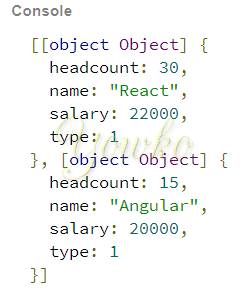## 找出所有符合條件的物件

• 陣列範例

``````var dataobj=[
{
name:'ASP.NET MVC',
type:3,
salary:15000,
},{
name:'Java',
type:3,
salary:25000,
},{
name:'Python',
type:3,
salary:22000,
},{
name:'React',
type:1,
salary:22000,
},{
name:'Angular',
type:1,
salary:20000,
},{
name:'Oracle',
type:2,
salary:28000,
},{
name:'Sql Server',
type:2,
salary:18000,
}
];
``````
1. 使用自定 function

• anonymous function

``````function findObjectByProporigin(arr, prop, val) {
let result=[];
arr.map(function(el){
if(el[prop]===val){
result.push(el);
}
});
return result;
}
findObjectByProporigin(dataobj,"type",3)
``````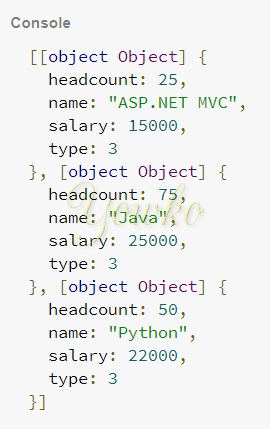• arrow function

``````function findObjectByProp(arr, prop, val) {
let result=[];
arr.map(el=>{
if(el[prop]===val){
result.push(el);
}
});
return result;
}
findObjectByProp(dataobj,"type",2)
``````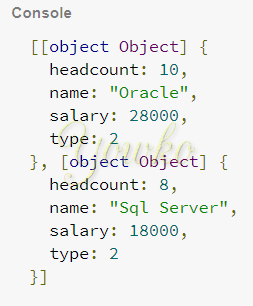2. 使用 ES6 - `filter`

``````dataobj.filter(obj =>obj.type === 3)
``````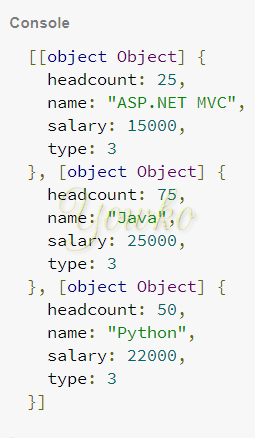3. 使用 lodash - `filter`

``````_.filter(dataobj, (obj)=>obj.type === 2)
``````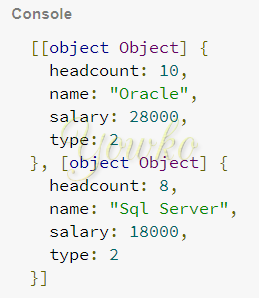4. 使用 Ramda - `filter`

``````R.filter(obj=>obj.type===1, dataobj)
``````